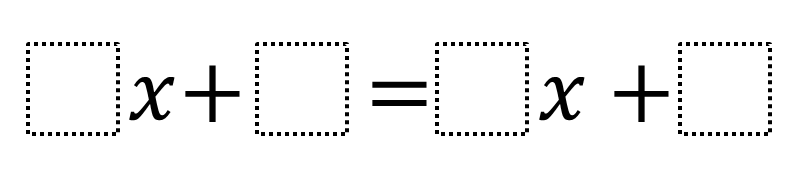# Create an Equation with a Solution Closest to Zero

Directions: Using the digits 1 to 9, at most one time each, fill in the boxes to create an equation such that the solution is as close to zero as possible.### Hint

Which kinds of numbers are closer to zero?
How does the digit you choose for the constant or coefficient affect the solution’s value?

There are many equations that will get to the correct answer of 1/8 or -1/8. Here are some possible equations examples:

9x + 3 = 1x + 4
1x + 7 = 9x + 8

Source: Daniel Luevanos

## Difference of Squares and Sum of Cubes

Directions: Using the digits 0 to 9 at most one time each, place a digit …

1.5x+2=4x+4

•I’m not sure you read the directions closely enough. First that solution is fairly far from zero. Additionally, you cannot reuse 4.

•9x + 3 = x + 2

•Anon is right

•If this is a question
5x+2=4x+4
_4. _4

X+2=4
_2. _2

X=2

•2x+4=x+8
-x -x
_________
1x+4=8
-4 -4
________
1x=4 x=4
1 1

•Nevaeh Smallwood

4x+2=5x+3

2.9x+3=1x + 4

3.1x+1=3x+2

4.I’m new to open middle, can you combine digits and use, for example 987x and 1x?

•No Scott, only one number per box.

5.Wouldn’t 1/9 or -1/9 be closer to 0 than 1/8 or -1/8? What about the equation 9x+2=0x+1?

•nevermind just re-read that it was 1-9 not 0-9. The desmos version includes the 0.

•Yeah, that one is similar but different.

•6.9x + 3 = x +2

7.9x + 3 = x + 2

8.9x+8=1x+7

9.7x – 4 = 3x – 6
Can we have a different equation from the problem across the equal sign ?

10.Oghenerukevwe Ayoro

8x + 4 = 5 – 2x

11.12.•You can only use each number once, you used 1 four times. Also, zero is not the only solution to yours.

13.9x+3=1x+4
1x+7=9x+8

14.15.1x+7= 9x+8

16.7x+4=5x+7

17.4x+6=2x+8

18.3x+8=9x+5

0.5

19.20.1x+1=3x+2

21.22.9x+3=1x+4

23.2x+4=4x+5

•I meant x+1= 2x+1.
I put the answer for a different question

24.2x+2/87816=3x+1/87816?

•3x+2.00000004=4x+2

25.26.27.6x+3=5x+2

28.x+4=9x+3
9x+3=x+2

29.1x + 7 = 9x + 8

30.9x+5=x+6

31.7x+8=5x+7

32.7x+1=2x+1.5

33.9x+5=x+4

34.9x+7=x+8

35.9x+3=1x+3

•9x + 3 = x +2

36.37.9x+1=x+6

38.9x+5=x+4

39.40.william hockstein

7x+.5=7x+.45

41.9x+7=x+8

42.10000x + 1 = x +2

43.250x+29=-750x+28

44.45.7x + 1 = 6x + 3

46.9x+1/4=10x+1/8

47.9x+4=x+5

48.6x+3=5x+2

49.7x+5=2x+4

50.2x +5 = 3x + 4.9

-x = -0.1

x= 0.1

51.9x+7=2x+8

52.1x+4=9x+3

53.2x+3=x+4

54.9x+2=x+1

55.•wait, how is 1/8 or -1/8 the closest to zero?

56.5x+2= 4x+4

57.x+1.1/4 = 2x+1 1/2

58.7x+1=2x+3

59.1x + 7 = 9x + 8

60.Create an Equation with a Solution Closest to Zero
Directions: Using the digits 1 to 9 at most one time each, place a digit in each box to create an equation such that the solution is as close to zero as possible.
_ x+ _ =_ x + _ -> original problem
3x +5 = 8x +6 -> my attempt

61.9x+3=1x + 4

62.J0\$hu@ g€|\$t

x+7=9x+8

63.9x+1=2x+3
9x-2x=3-1
7x=2
x=2/7
x=0,28

64.1x + 2 = 3x + 4

65.Untuneable Instrument

8x+1=3x+2

5x+1

x=0.2

66.987654x + 2 = 1x + 3

x=.0000010125…

Had the question been worded “fill in each box with one digit,” then the correct answer would have been something like

9x + 2 = 1x + 3
x = 1/8

•I like the way you think. Well done. We as math teachers should be aware when the directions are not clear.

67.9x + 7 = 1x + 8

8x = 1

x = 1/8